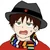## FANDOM

25,509 Pages# DemonGodMitchAubin

## aka Mitchell

• I live in Heaven and Hell Simultaneously
• I was born on August 22
• My occupation is You Know... Nerdy Stuff
• I am Male
•## FE3H Dodging Calcs

April 4, 2020 by DemonGodMitchAubin

In Fire Emblem Three Houses, the characters are able to dodge both Meteors and the Immaculate One's Light right as it is above their head, so I will find out what that results in. Everyone dodges the same distance whenever they dodge an attack, so I will use Dimitri's dodge animation to get an average distance moved. I'll also do a re-calc of dodging bolting just so we can have a Three Houses version.

Dimitri's Height = 1.88 Meters = 949 px

1.88 Meters/949 px = 0.00198 Meters per px

Distance Moved = 594.12 px = 1.18 Meters

The characters don't begin to dodge until the spell is right above the head of the person, so I'll lowball to 1 Foot, which is 0.3048 Meters

Calculation = ((Distance the character moved in Meters)*(Speed of Attack))/(Distance…

•## FDKM Natsu Splits Ikusa-Tsunagi's Clouds

April 2, 2020 by DemonGodMitchAubin

In Fairy Tail chapter 434 to 435, Natsu destroys Ikusa-Tsunagi and splits his cloud's in the process as well. The cloud was split towards the horizon, so that means the distance is 20,000 Meters. The cloud was never specified whether or not it was split omnidirectionally, so I'll do a Low-End and High-End for both scenarios.

Distance = 20,000 Meters

Average height of Storm Clouds is 8000 Meters.

Volume = pi*(viewing distance)^2*(cloud thickness)

Volume = pi*(20,000^2)*(8000 M) = 1.0053096e+13

So then we multiply the volume by 1.003 to get Mass

1.0053096e+13*1.003 = 1.0083256e+13

The Cloud Split took 1 Panel, so I'll assume a timeframe of 5 Seconds.

20,000 Meters/5 Seconds = 4000 m/s

Omnidirectionally:

KE = (1/12)*Cloud Mass*(Speed of Cloud Movement)…

•## Ren Tao cuts a car in half

March 30, 2020 by DemonGodMitchAubin

Ren Tao cuts a car in half. The area cut was basically 2 rectangles, I'll also assume a 75% hollowness of the car's area, since we don't have a full shot of the car.

Ren Tao = 1.45 Meters = 84.61 px

1.45 Meters/84.61 px = 0.017 Meters per px

Length of Car Back = 184.53 px = 3.14 Meters

Height of Car Back = 119.52 px = 2.03 Meters

Area of Car Back = 6.3742 Meters^2

Length of Car Front = 54.15 px = 0.92 Meters

Height of Car Front = 71.25 px = 1.21 Meters

Area of Car Front = 1.11 Meters^2

Full Area of Area Cut = 7.4874 Meters^2

Assuming 75% Hollowness, the are we'll use is 1.87 Meters^2

The formula is (Compresive Strength*Area*Width of the blade)

Area = 1.87 Meters^2

The width of weapon blades is 0.1 Millimeters wide.

The average compresive strength of all…

•## Dragon Quest 11 Calcs

March 29, 2020 by DemonGodMitchAubin

I was asked to calc some feats for Dragon Quest 11, so let's do them.

Krystalinda creates a winter storm that covers the entirety of Sniflheim

Photo 1:

Ship Height = 4.58 Meters = 301.01 px

4.58 Meters/301.01 px = 0.015 Meters per px

Distance Moved = 1252 px = 19 Meters

The time it moved this distance was 0.4 Seconds

Velocity = 19 Meters/0.4 Seconds = 47.5 m/s

KE = 0.5*Mass*Velocity^2

KE = 0.5*(107002.5 Kg)*(47.5 m/s)^2 = 0.02 Tons (Small Building level)

This calc is pretty janky and hella lowballed, but I was asked to try it.

•## NNT Mael disperses some clouds

March 22, 2020 by DemonGodMitchAubin

This is a revision of Mael's Cloud feat, where he disperses the Original Demon's clouds omnidirectionally. This is based on M3X's version of the calc, and he asked me to make a new blog for the revision, but I'm still gonna give him most of the credit.

Using this calc for the size of the Country, we know the distance is 900km. Thats the diameter, now we need to find the distance where the storm went.

Red Line = 900 km = 400 px

900 km/400 px = 2.25 km per px

Blue Line = 187 px = 420.75 km

The clouds height's are 9.9 km

Mass = 5.5196816e+15 kg

We will use 30 Seconds like with before

Velocity = 420750 m/30 s = 14025 m/s

KE = (1/12)*Cloud Mass*(Speed of Cloud Movement)^2

KE = (1/12)*5.5196816e+15*(14025)^2 = 21.6 Teratons (Country leve…